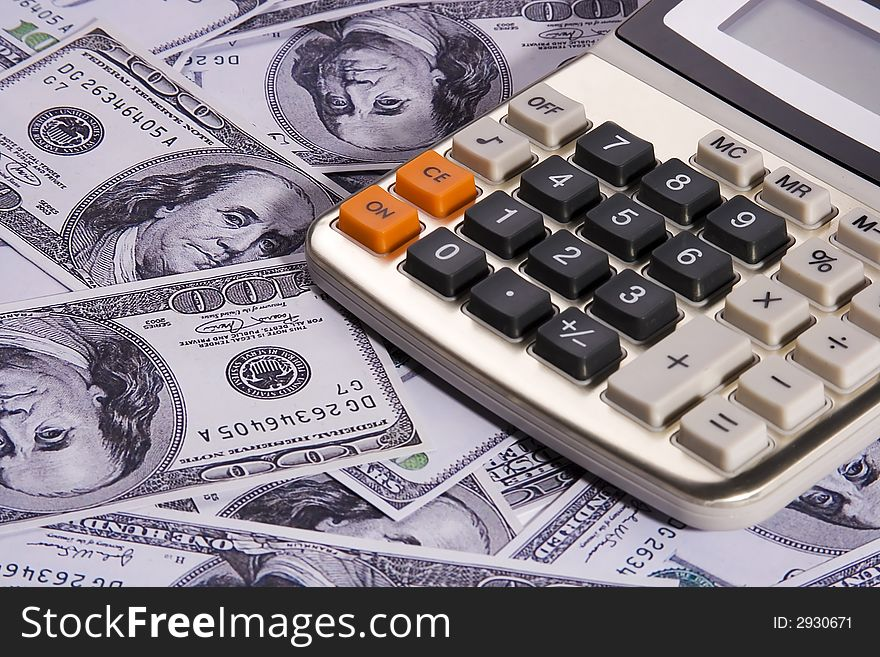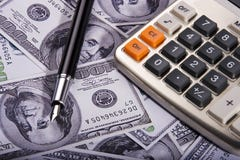Calculator Over Money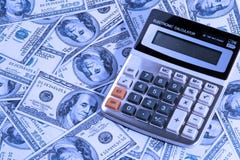Calculator Over Money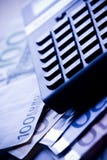CalculatorCalculator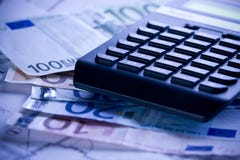Calculator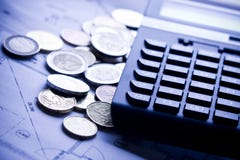Calculator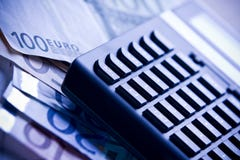Calculator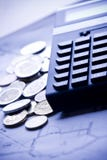Calculator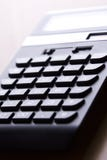Calculator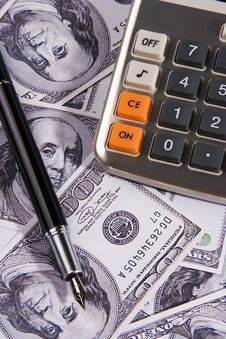Calculator Over Money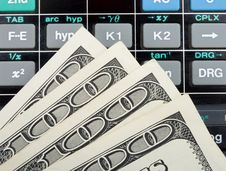Dollars Over Calculator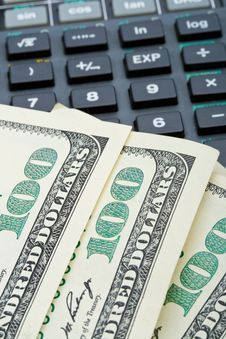Many Dollars Over Calculator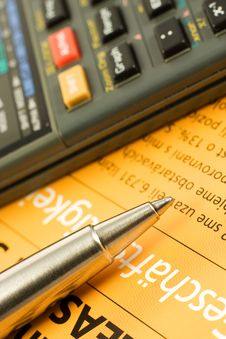Calculator Over Orange Paper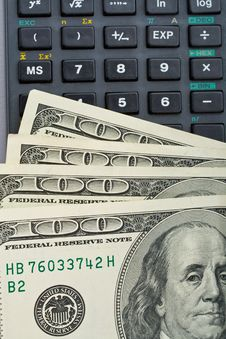Many Us Dollars Over Calculator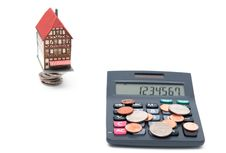House With Coins And Calculator Over White Backgro